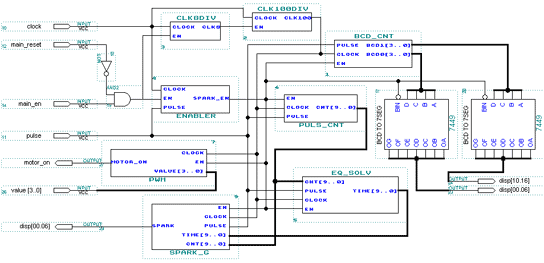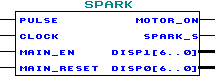## 2.2. The Spark Generator in VHDL

### 2.2.1. The components

The basic components used for the VHDL applications an enabler, to enable or not the others a pulse counter which counts the number of clock cycles between each a solver for the equation, to calculate when the spark must a spark generator, which generates the spark when a PWM generator to modulate the speed of the engine from the a BCD counter, used for the two 7 segment displays and evaluate the speed.

The clock used is a 8 Mhz clock, so we use 2 clock dividers, to obtain the appropriate clock rate, which is 10 Khz.

### 2.2.2. The main component

All the VHDL components created are merged in one main file which is represented by this schematic:

Spark generator: symbolic representationFinally the component uses 4 input pins and 16 output pins.

##### [ Contents | Previous Page | Next Page ]
For any comment/Pour tout commentaire : stephane@odul.net